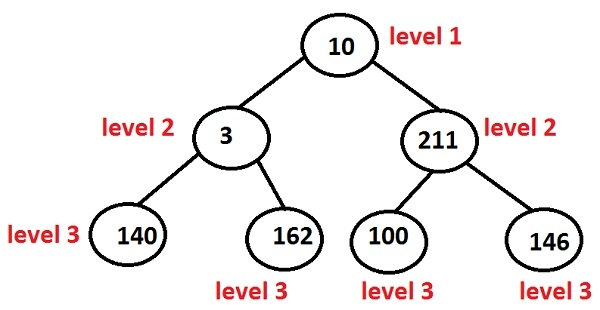# Print Levels of all nodes in a Binary Tree in C++ Programming.

Given the binary tree, the task is to print the level associated with every key stored in a node starting from 1 to nIn the above tree, nodes are −

10 at level 1
3 and 211 at level 2
140, 162, 100 and 146 at level 3

Given the key the program must print the level of that particular key.

## Example

Input: 10 3 211 140 162 100 146
Output:
level of 10 is 1
Level of 3 is 2
Level of 211 is 2
Level of 140 is 3
Level of 162 is 3
Level of 100 is 3
Level of 146 is 3

## Algorithm

START
Step 1 -> create a structure of a node as
struct node
struct node *left, *right
int data
End
Step 2 -> function to create a node
node* newnode(int data)
node *temp = new node
temp->data = data
temp->left = temp->right= NULL
return temp
step 3 -> create function for finding levels of a node
void levels(Node* root)
IF root=NULL
Return
End
Create STL queue<pair<struct Node*, int> >que
que.push({root, 1})
create STL pair<struct Node*, int> par
Loop While !que.empty()
par = que.front()
que.pop()
print par.first->data and par.second
IF par.first->left
que.push({ par.first->left, par.second + 1 })
END
IF par.first->right
que.push({ par.first->right, par.second + 1 })
End
End
STOP

## Example

Live Demo

#include <bits/stdc++.h>
using namespace std;
//structure of a node
struct Node{
int data;
struct Node *left, *right;
};
//it will print levels of a tree
void levels(Node* root){
if (root==NULL)
return;
queue<pair<struct Node*, int> >que;
que.push({root, 1});
pair<struct Node*, int> par;
while (!que.empty()) {
par = que.front();
que.pop();
cout << "Level of " << par.first->data << " is " << par.second << "\n";
if (par.first->left)
que.push({ par.first->left, par.second + 1 });
if (par.first->right)
que.push({ par.first->right, par.second + 1 });
}
}
//function to create nodes annd hence generate tree
Node* newnode(int data){
Node* temp = new Node;
temp->data = data;
temp->left = temp->right = NULL;
return temp;
}
int main(){
Node* root = NULL;
//it will create a node
root = newnode(34);
root->left = newnode(12);
root->right = newnode(50);
root->left->left = newnode(11);
root->left->right = newnode(54);
levels(root);
return 0;
}

## Output

if we run the above program then it will generate the following output

Level of 34 is 1
Level of 12 is 2
Level of 50 is 2
Level of 11 is 3
Level of 54 is 3